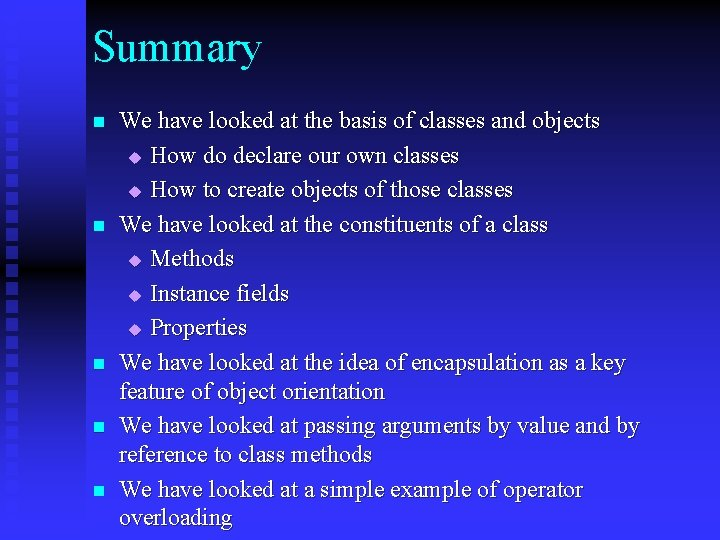# Object Oriented Programming Classes and Objects Dr Mike

• Slides: 58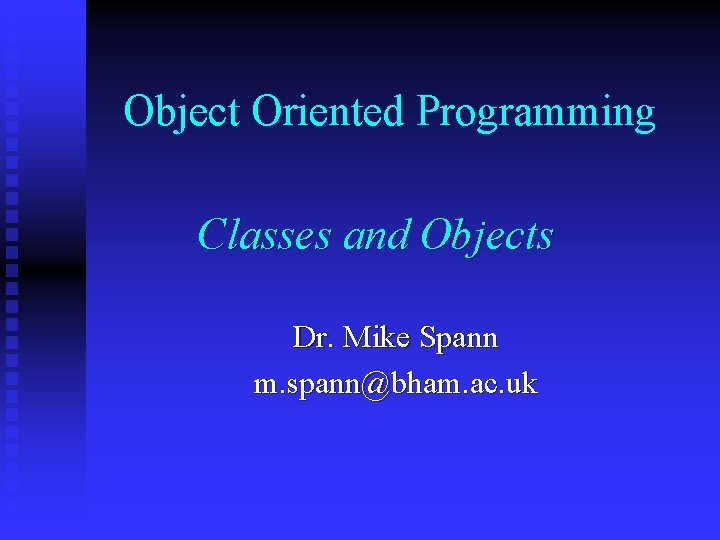Object Oriented Programming Classes and Objects Dr. Mike Spann m. [email protected] ac. uk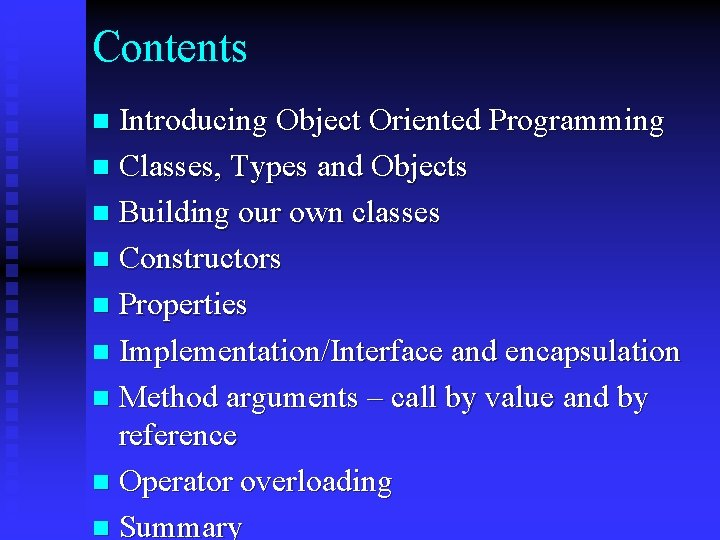Contents Introducing Object Oriented Programming n Classes, Types and Objects n Building our own classes n Constructors n Properties n Implementation/Interface and encapsulation n Method arguments – call by value and by reference n Operator overloading n Summary n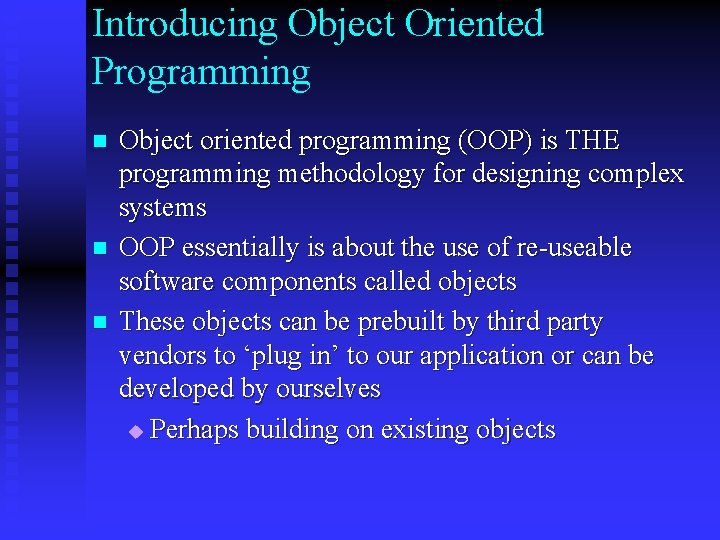Introducing Object Oriented Programming n n n Object oriented programming (OOP) is THE programming methodology for designing complex systems OOP essentially is about the use of re-useable software components called objects These objects can be prebuilt by third party vendors to ‘plug in’ to our application or can be developed by ourselves u Perhaps building on existing objectsIntroducing Object Oriented Programming n There are good reasons why building our application out of such objects has major advantages u We can abstract out the complex state and behaviour of individual objects u Only the interface between the objects in our application is important u We can use prebuilt objects such as FCL objects t We are able to extend these objects to meet our own needs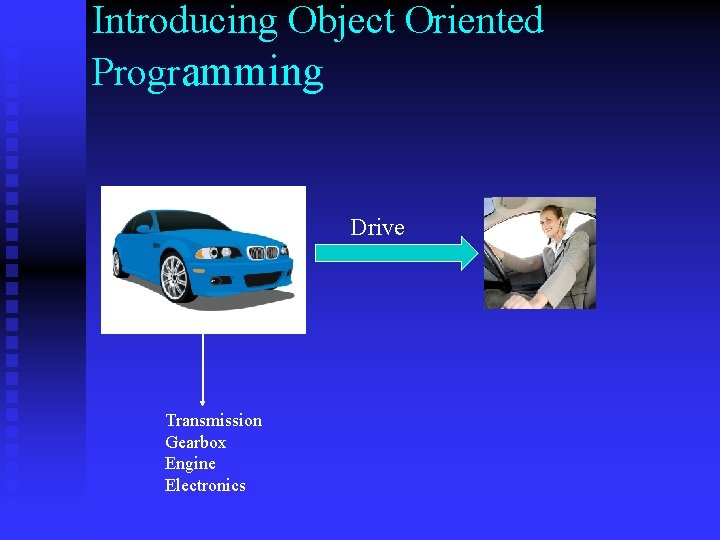Introducing Object Oriented Programming Drive Transmission Gearbox Engine ElectronicsClasses, Types and Objects The FCL consists of classes, interfaces structures and enumerated types u Collectively known as types u We have already seen some examples of classes t System, Console, Form t Classes are reference types t Value types are known as structures n We can create our own classes, interfaces, structures and enumerated types n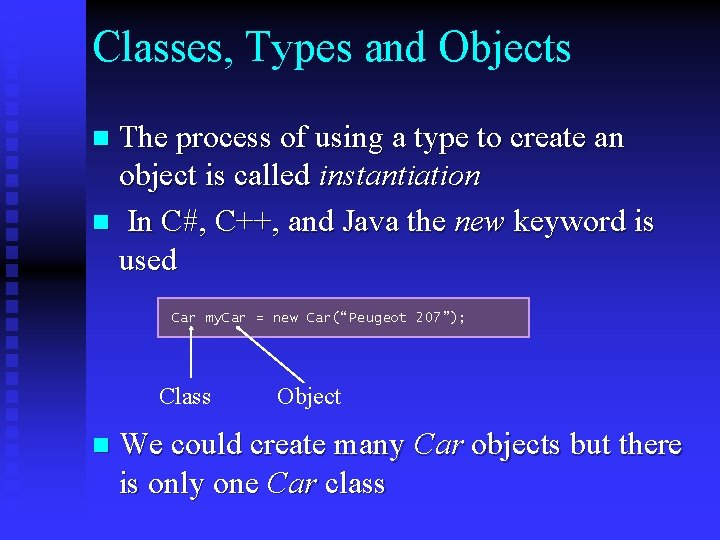Classes, Types and Objects The process of using a type to create an object is called instantiation n In C#, C++, and Java the new keyword is used n Car my. Car = new Car(“Peugeot 207”); Class n Object We could create many Car objects but there is only one Car class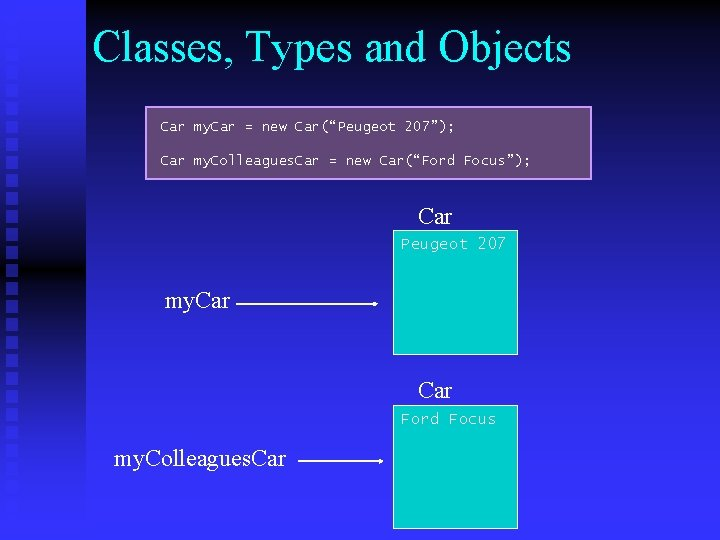Classes, Types and Objects Car my. Car = new Car(“Peugeot 207”); Car my. Colleagues. Car = new Car(“Ford Focus”); Car Peugeot 207 my. Car Ford Focus my. Colleagues. Car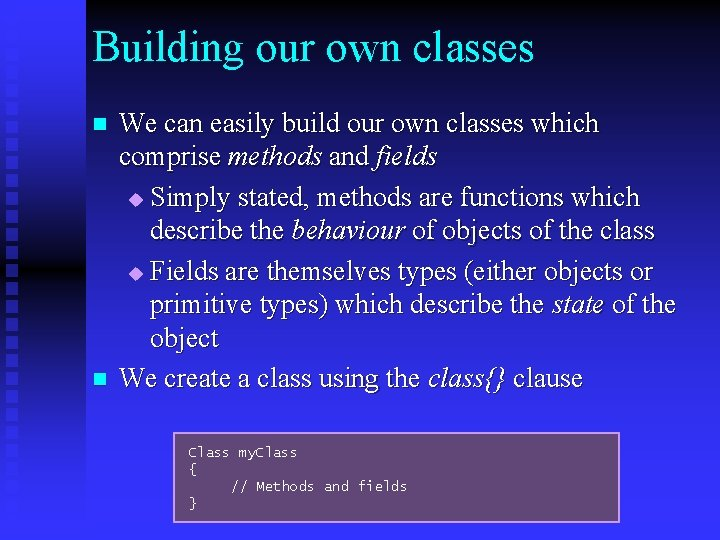Building our own classes n n We can easily build our own classes which comprise methods and fields u Simply stated, methods are functions which describe the behaviour of objects of the class u Fields are themselves types (either objects or primitive types) which describe the state of the object We create a class using the class{} clause Class my. Class { // Methods and fields }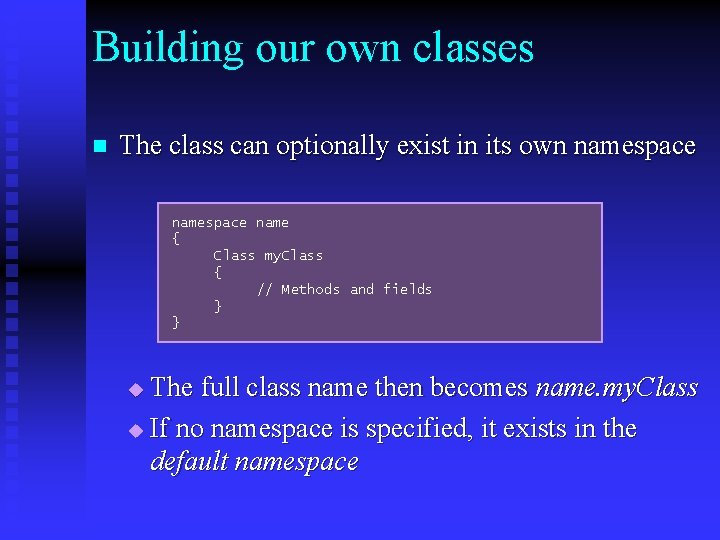Building our own classes n The class can optionally exist in its own namespace name { Class my. Class { // Methods and fields } } The full class name then becomes name. my. Class u If no namespace is specified, it exists in the default namespace u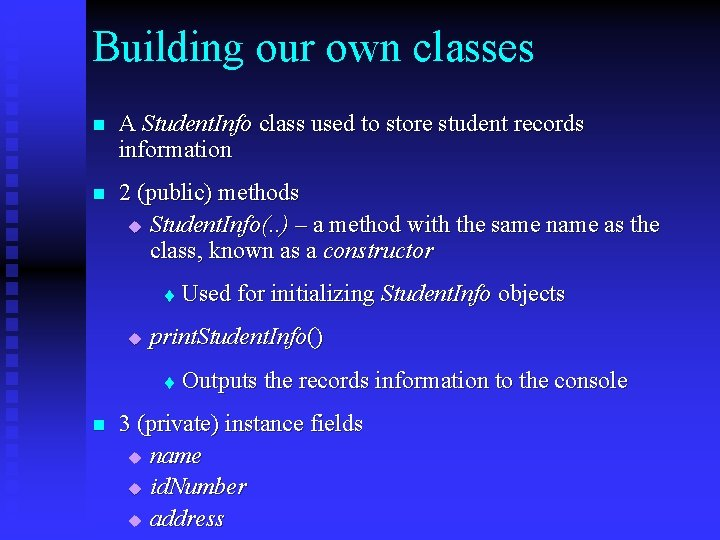Building our own classes n A Student. Info class used to store student records information n 2 (public) methods u Student. Info(. . ) – a method with the same name as the class, known as a constructor t u print. Student. Info() t n Used for initializing Student. Info objects Outputs the records information to the console 3 (private) instance fields u name u id. Number u address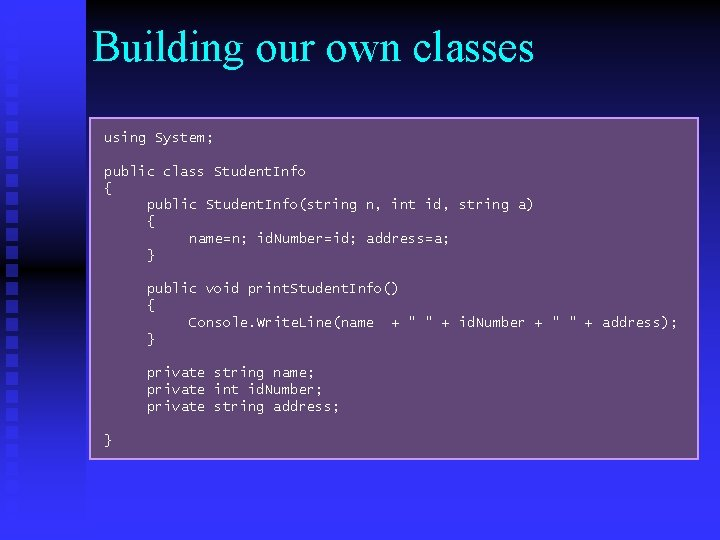Building our own classes using System; public class Student. Info { public Student. Info(string n, int id, string a) { name=n; id. Number=id; address=a; } public void print. Student. Info() { Console. Write. Line(name + " " + id. Number + " " + address); } private string name; private int id. Number; private string address; }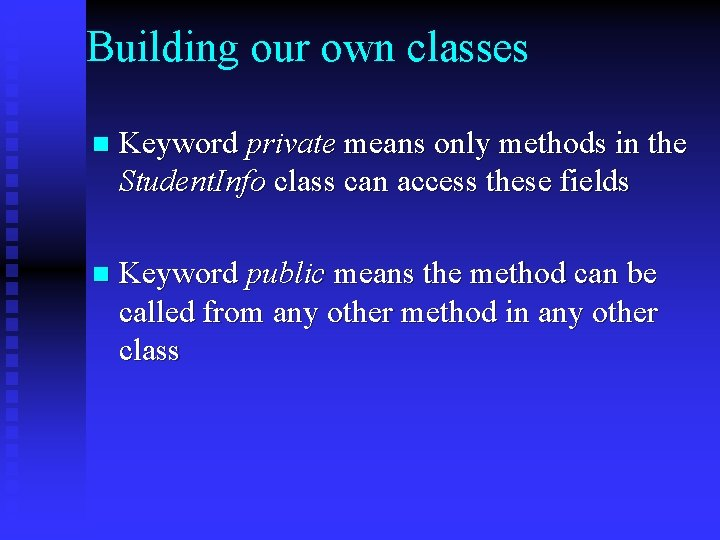Building our own classes n Keyword private means only methods in the Student. Info class can access these fields n Keyword public means the method can be called from any other method in any other class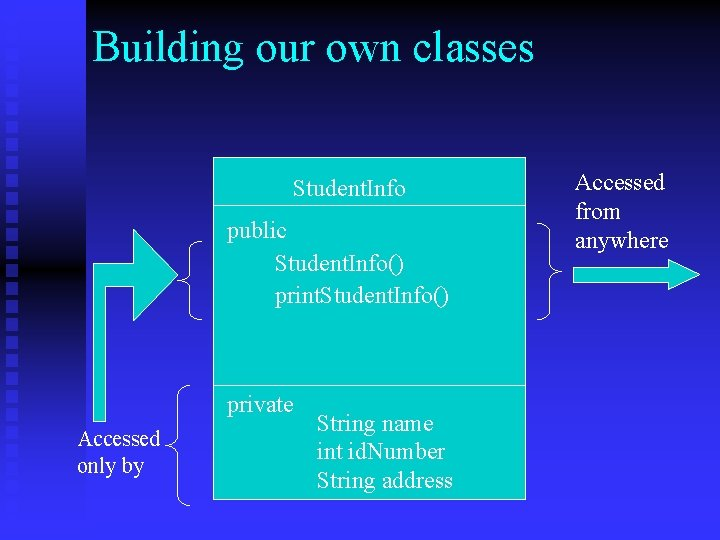Building our own classes Student. Info public Student. Info() print. Student. Info() private Accessed only by String name int id. Number String address Accessed from anywhere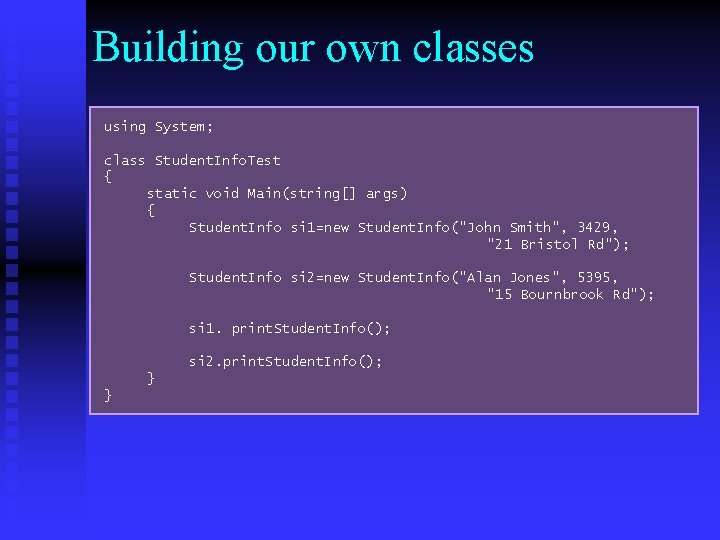Building our own classes using System; class Student. Info. Test { static void Main(string[] args) { Student. Info si 1=new Student. Info("John Smith", 3429, "21 Bristol Rd"); Student. Info si 2=new Student. Info("Alan Jones", 5395, "15 Bournbrook Rd"); si 1. print. Student. Info(); si 2. print. Student. Info(); } }Building our own classes So far in the Student. Info class, we have 3 private instance fields u name u id. Number u address n These instance fields are known as nonstatic instance fields u Each Student. Info object has its own separate copy of these three fields nBuilding our own classes n We can also have static instance fields which represent class wide information u For example we might want to keep the total number of students registered as a static field of Student. Info u Not sensible to keep this in each Student. Info object t Can be accessed by methods of Student. Info t But not vice versa – non-static methods cannot access static instance fields! t Can be get and set using static properties in the usual waypublic class Student. Info { public Student. Info(string n, int id, string a) { name=n; id. Number=id; address=a; } public void print. Student. Info() { Console. Write. Line(name + " " + id. Number + " " + address); Console. Write. Line(number. Registered + " students “); } // Name, Address and ID properties. . . . public static int Number. Registered { get { return number. Registered; } set { number. Registered = value; } } private } static int number. Registered; string name; int id. Number; string address;Building our own classes class Student. Info. Test { static void Main(string[] args) { Student. Info si 1=new Student. Info("John Smith", 3429, "21 Bristol Rd"); Student. Info si 2=new Student. Info("Alan Jones", 5395, "15 Bournbrook Rd"); Student. Info. Number. Registered = 2; si 1. print. Student. Info(); } }Building our own classes class Student. Info private String name; private int id. Number; private String address private static number. Registered=2; Student. Info s 1=new Student. Info(. . ); Student. Info s 2=new Student. Info(. . ); name=“John Smith" id. Number=3429 address= "21 Bristol Rd" s 1 name=“Alan Jones“ id. Number= 5395 address="15 Bournbrook Rd" s 2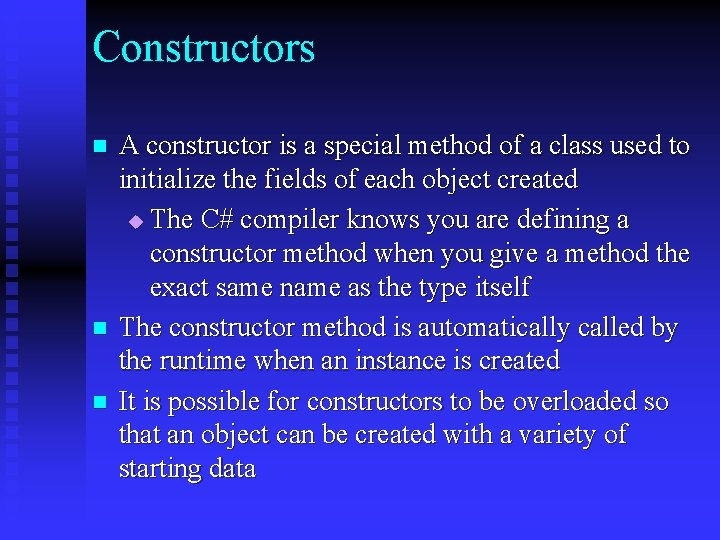Constructors n n n A constructor is a special method of a class used to initialize the fields of each object created u The C# compiler knows you are defining a constructor method when you give a method the exact same name as the type itself The constructor method is automatically called by the runtime when an instance is created It is possible for constructors to be overloaded so that an object can be created with a variety of starting data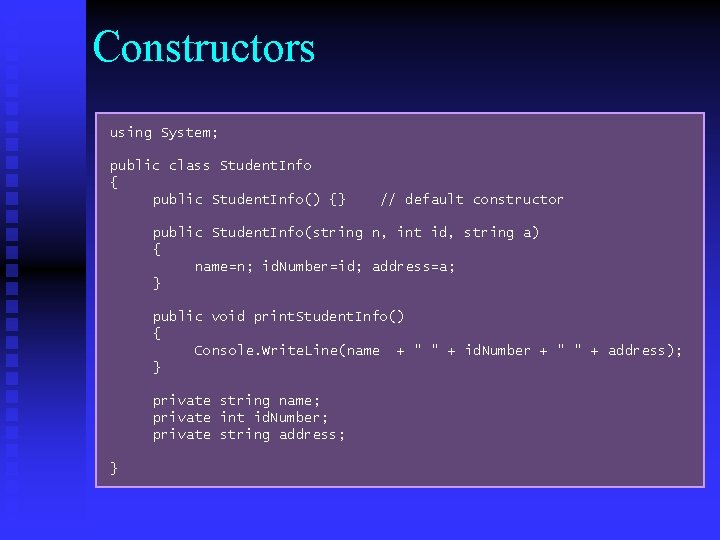Constructors using System; public class Student. Info { public Student. Info() {} // default constructor public Student. Info(string n, int id, string a) { name=n; id. Number=id; address=a; } public void print. Student. Info() { Console. Write. Line(name + " " + id. Number + " " + address); } private string name; private int id. Number; private string address; }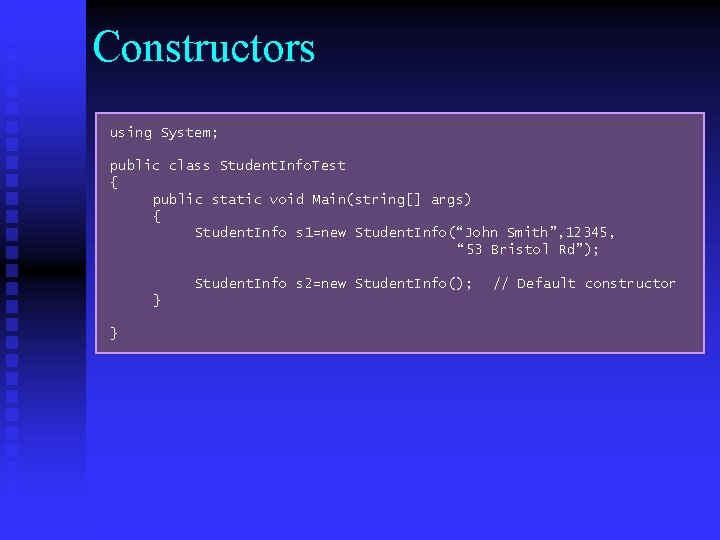Constructors using System; public class Student. Info. Test { public static void Main(string[] args) { Student. Info s 1=new Student. Info(“John Smith”, 12345, “ 53 Bristol Rd”); Student. Info s 2=new Student. Info(); } } // Default constructor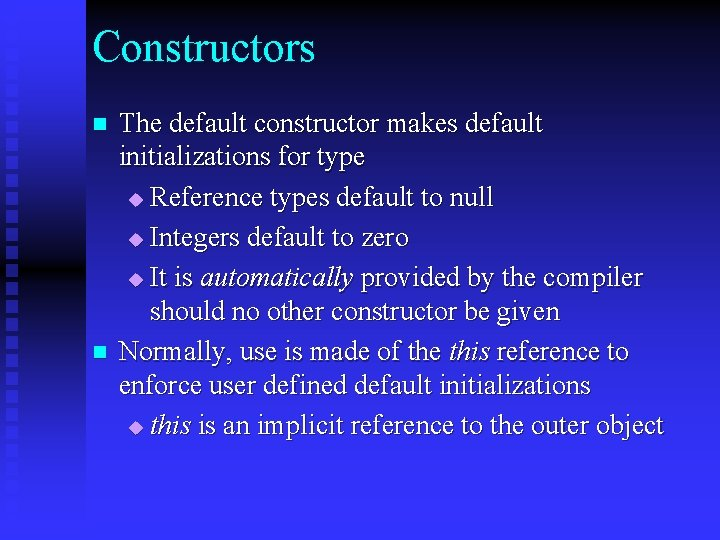Constructors n n The default constructor makes default initializations for type u Reference types default to null u Integers default to zero u It is automatically provided by the compiler should no other constructor be given Normally, use is made of the this reference to enforce user defined default initializations u this is an implicit reference to the outer objectConstructors Student. Info public Student. Info( this ) print. Student. Info( private String name int id. Number String address ) thisConstructors using System; public class Student. Info { public Student. Info() {this(“No name”, 0, “Birmingham University”} public Student. Info(string n, int id, string a) { name=n; id. Number=id; address=a; } public void print. Student. Info() { Console. Write. Line(name + " " + id. Number + " " + address); } private string name; private int id. Number; private string address; }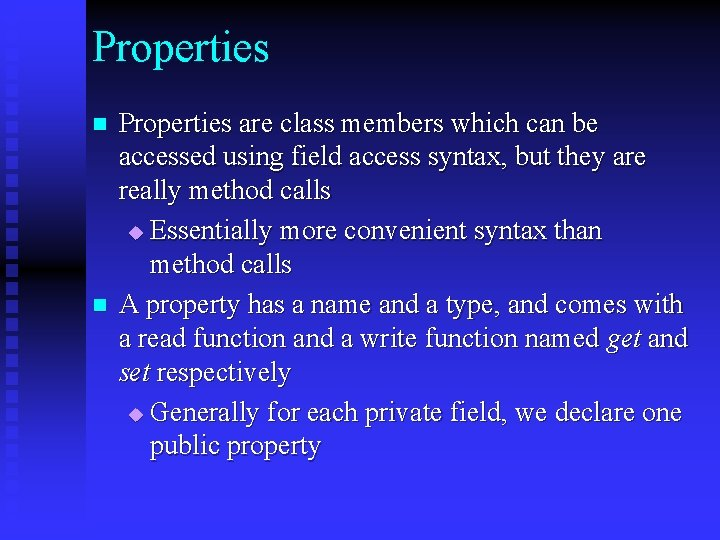Properties n n Properties are class members which can be accessed using field access syntax, but they are really method calls u Essentially more convenient syntax than method calls A property has a name and a type, and comes with a read function and a write function named get and set respectively u Generally for each private field, we declare one public propertypublic class Student. Info { public Student. Info(string n, int id, string a) { name=n; id. Number=id; address=a; } public void print. Student. Info() { Console. Write. Line(name + " " + id. Number + " " + address); } public string Name { get { return name; } set { name = value; } } public string Address { get { return address; } set { address = value; } } public int ID { get { return id. Number; } set { id. Number = value; } } private string name; private int id. Number; private string address; }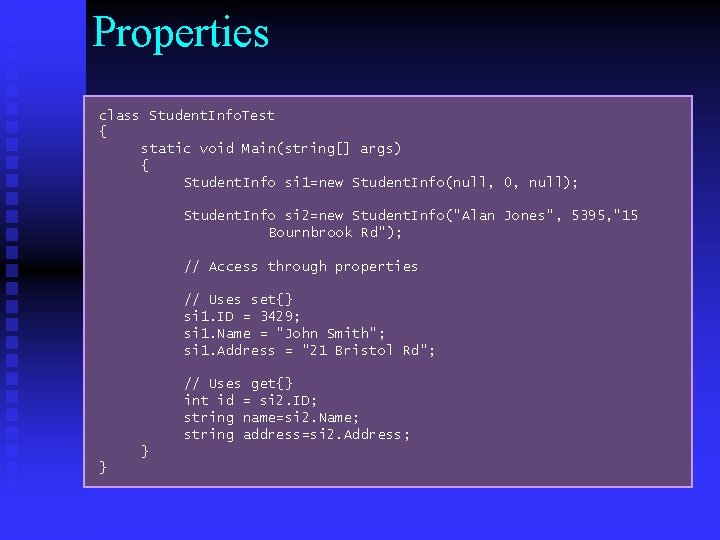Properties class Student. Info. Test { static void Main(string[] args) { Student. Info si 1=new Student. Info(null, 0, null); Student. Info si 2=new Student. Info("Alan Jones", 5395, "15 Bournbrook Rd"); // Access through properties // Uses set{} si 1. ID = 3429; si 1. Name = "John Smith"; si 1. Address = "21 Bristol Rd"; // Uses get{} int id = si 2. ID; string name=si 2. Name; string address=si 2. Address; } }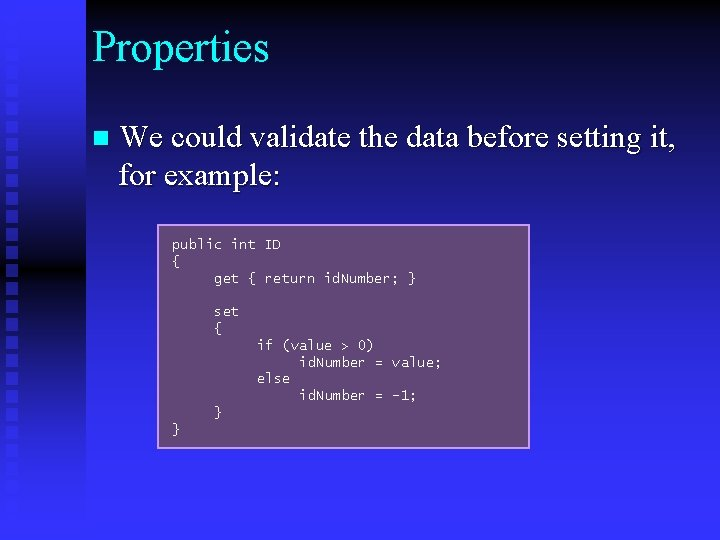Properties n We could validate the data before setting it, for example: public int ID { get { return id. Number; } set { if (value > 0) id. Number = value; else id. Number = -1; } }Implementation/Interface and encapsulation n Generally, instance fields are private u They represent the implementation of the class (or the object internal state) Methods are generally public u They represent the interface to the class u The define how objects can be used The separation of a class into public/private is a key feature of object-oriented programming u Sometimes known as encapsulationImplementation/Interface and encapsulation n The implementation of a class is specified in the private instance fields u They are hidden from outside the class n The interface to the class is specified by the public methods u They can be accessed from anywhere n The implementation may change but the interface must stay the same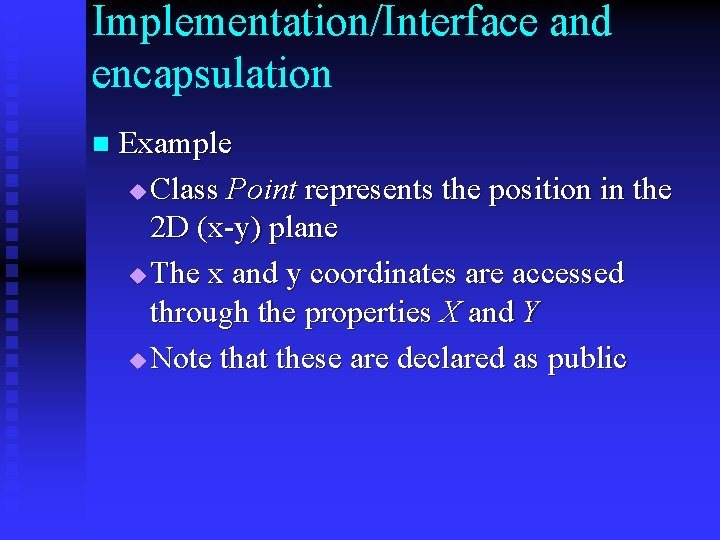Implementation/Interface and encapsulation n Example u Class Point represents the position in the 2 D (x-y) plane u The x and y coordinates are accessed through the properties X and Y u Note that these are declared as publicImplementation/Interface and encapsulation public class Point { public Point(int x, int y) { xpos = x; ypos = y; } public int X { get{ return xpos; } set { xpos=value; } } public int Y { get{ return ypos; } set { ypos=value; } } private int xpos, ypos; }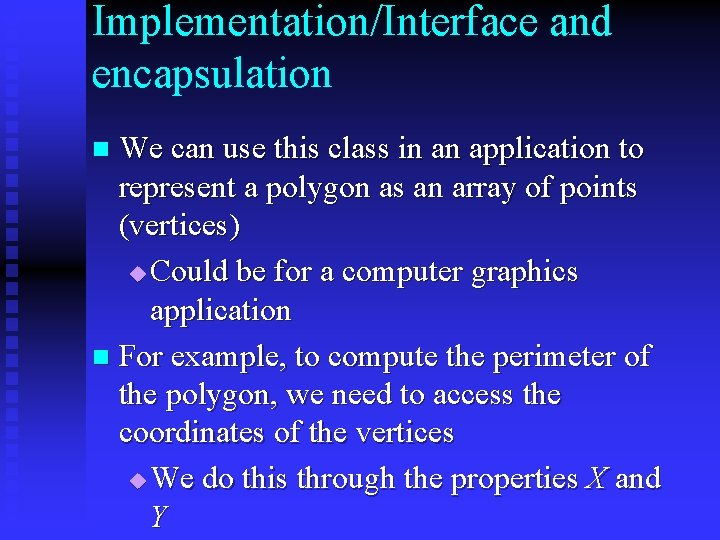Implementation/Interface and encapsulation We can use this class in an application to represent a polygon as an array of points (vertices) u Could be for a computer graphics application n For example, to compute the perimeter of the polygon, we need to access the coordinates of the vertices u We do this through the properties X and Y nImplementation/Interface and encapsulation class Polygon { public Polygon() {…} public double perimeter() { double pm=0; int nn; for (int n=1; n<num. Vertices; n++) { nn=n%num. Vertices; // Access vertices coordinates through properties int xv 1= vertices[n-1]. X; int yv 1=vertices[n-1]. Y; int xv=vertices[nn]. X; int yv=vertices[nn]. Y; pm+=(Math. sqrt((xv 1 -xv)*(xv 1 -xxv)+(yv 1 -yv)*(yv 1 -yv))); } return pm; } private Point[] vertices; private int num. Vertices; }Implementation/Interface and encapsulation n n The idea behind encapsulation is that changing the implementation of Point will not change the implementation of the Polygon class because Point objects are accessed through public properties or methods u The users of Point do not even need to know about it u Point is a re-useable software component Example – we can implement Point objects using a polar coordinate representation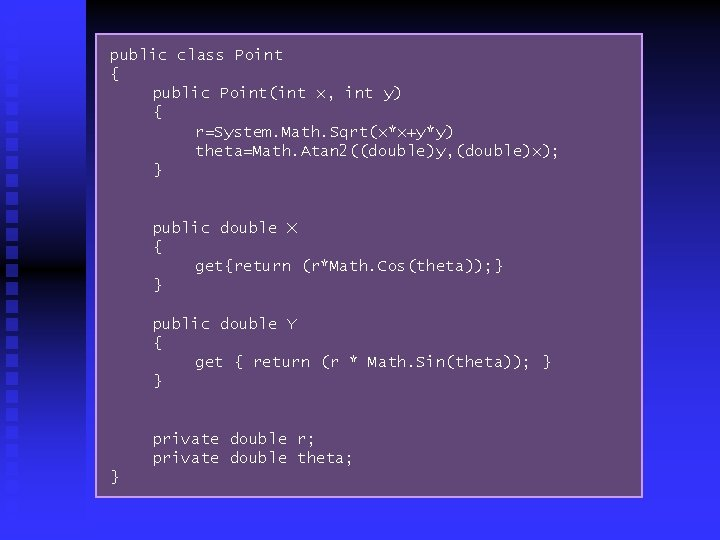public class Point { public Point(int x, int y) { r=System. Math. Sqrt(x*x+y*y) theta=Math. Atan 2((double)y, (double)x); } public double X { get{return (r*Math. Cos(theta)); } } public double Y { get { return (r * Math. Sin(theta)); } } private double r; private double theta; }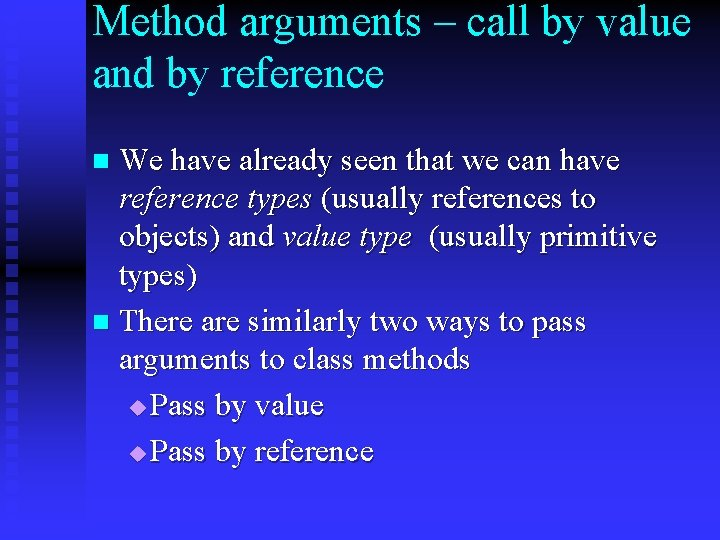Method arguments – call by value and by reference We have already seen that we can have reference types (usually references to objects) and value type (usually primitive types) n There are similarly two ways to pass arguments to class methods u Pass by value u Pass by reference n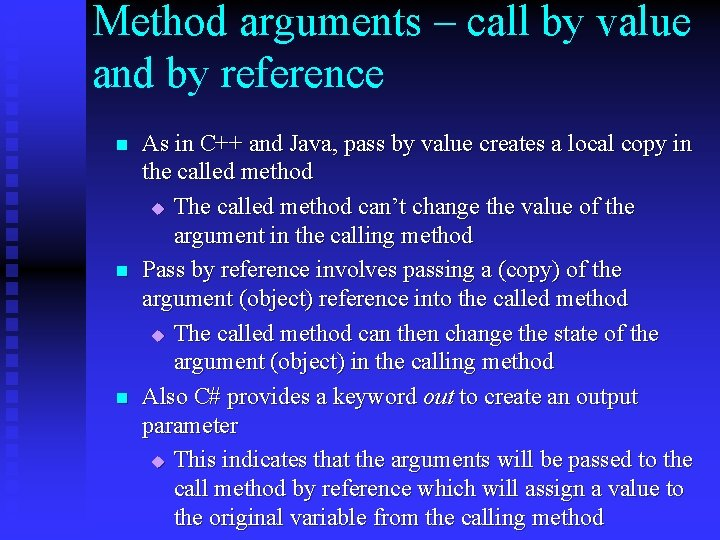Method arguments – call by value and by reference n n n As in C++ and Java, pass by value creates a local copy in the called method u The called method can’t change the value of the argument in the calling method Pass by reference involves passing a (copy) of the argument (object) reference into the called method u The called method can then change the state of the argument (object) in the calling method Also C# provides a keyword out to create an output parameter u This indicates that the arguments will be passed to the call method by reference which will assign a value to the original variable from the calling methodMethod arguments – call by value and by reference public class Square { public void call. By. Val(int x) { x = x * x; } public void call. By. Ref(ref int x) { x = x * x; } public void call. By. Out(out int x) { x = 3; x = x * x; } }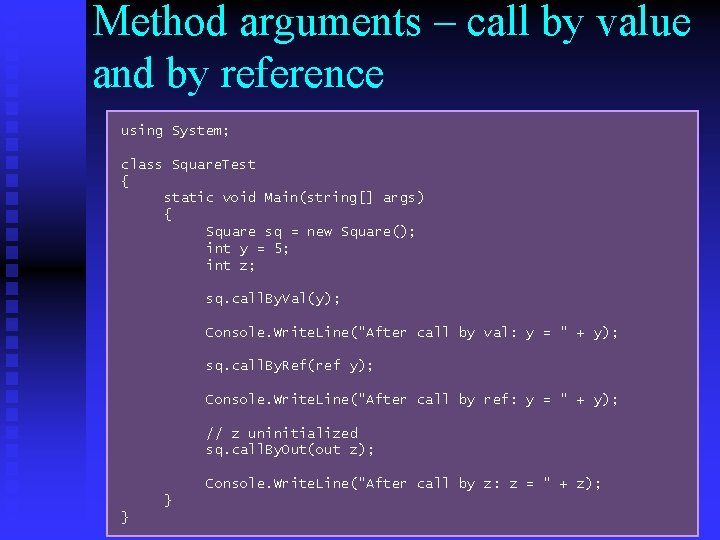Method arguments – call by value and by reference using System; class Square. Test { static void Main(string[] args) { Square sq = new Square(); int y = 5; int z; sq. call. By. Val(y); Console. Write. Line("After call by val: y = " + y); sq. call. By. Ref(ref y); Console. Write. Line("After call by ref: y = " + y); // z uninitialized sq. call. By. Out(out z); Console. Write. Line("After call by z: z = " + z); } }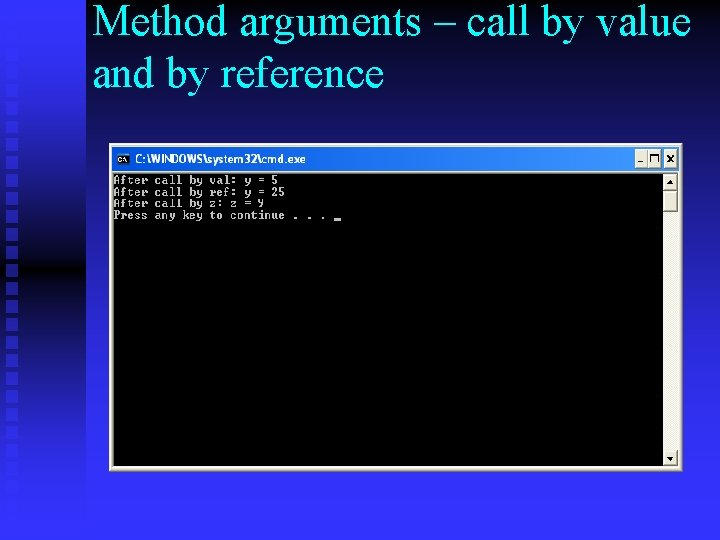Method arguments – call by value and by referenceMethod arguments – call by value and by reference n When we pass objects into methods, we are passing the object reference u But the object reference is normally passed by value u In other words we are passing a copy of the reference into the method u The object in the calling method can still be altered in the called method t But the reference in the calling method can’t be altered – it always refers to the same object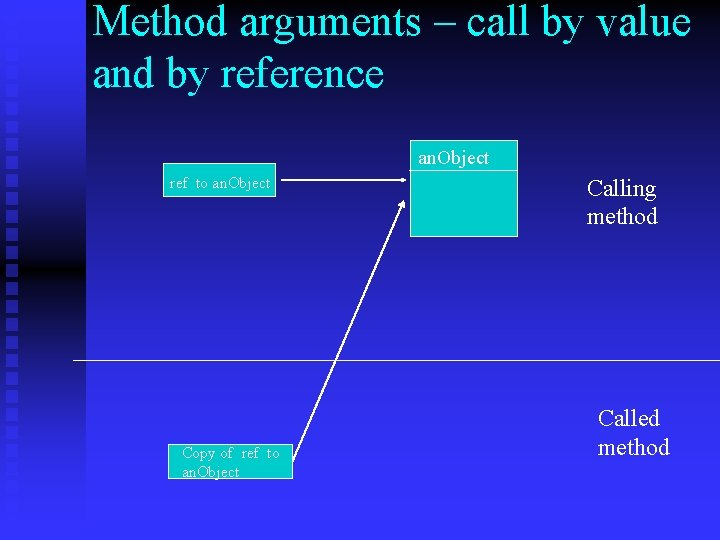Method arguments – call by value and by reference an. Object ref to an. Object Copy of ref to an. Object Calling method Called method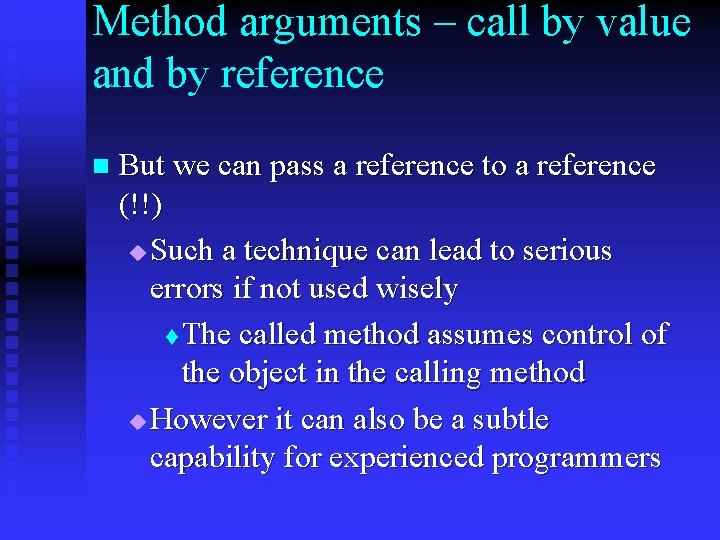Method arguments – call by value and by reference n But we can pass a reference to a reference (!!) u Such a technique can lead to serious errors if not used wisely t The called method assumes control of the object in the calling method u However it can also be a subtle capability for experienced programmers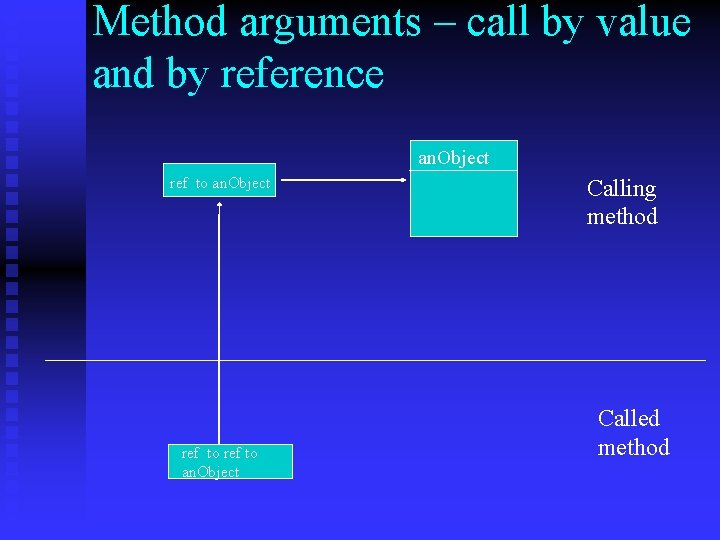Method arguments – call by value and by reference an. Object ref to an. Object Calling method Called method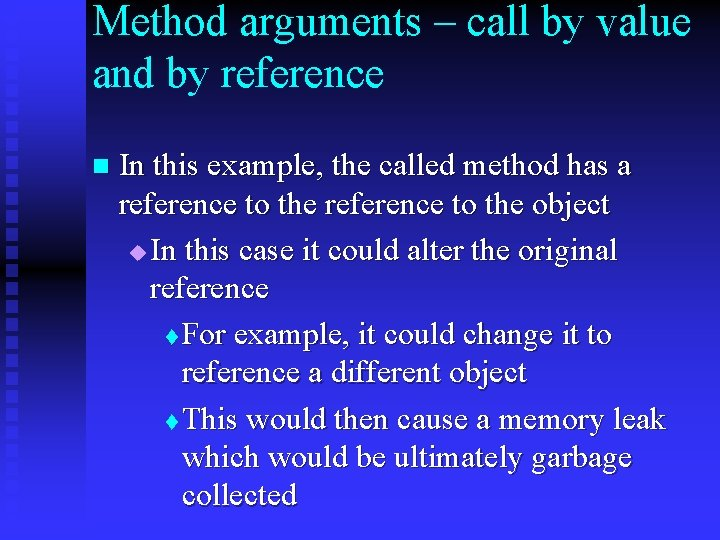Method arguments – call by value and by reference n In this example, the called method has a reference to the object u In this case it could alter the original reference t For example, it could change it to reference a different object t This would then cause a memory leak which would be ultimately garbage collectedMethod arguments – call by value and by reference public class my. Student. Records. Application { public static void set. IDpass. By. Val(Student. Info s) { s. ID = 9999; s = new Student. Info("no name", 0, "no fixed abode"); } public static void set. IDpass. By. Ref(ref Student. Info s) { s. ID = 9999; s = new Student. Info("no name", 0, "no fixed abode"); } }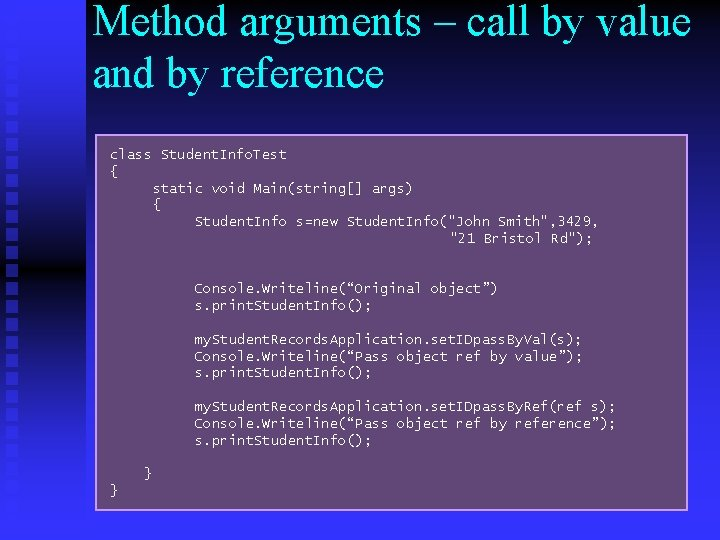Method arguments – call by value and by reference class Student. Info. Test { static void Main(string[] args) { Student. Info s=new Student. Info("John Smith", 3429, "21 Bristol Rd"); Console. Writeline(“Original object”) s. print. Student. Info(); my. Student. Records. Application. set. IDpass. By. Val(s); Console. Writeline(“Pass object ref by value”); s. print. Student. Info(); my. Student. Records. Application. set. IDpass. By. Ref(ref s); Console. Writeline(“Pass object ref by reference”); s. print. Student. Info(); } }Method arguments – call by value and by reference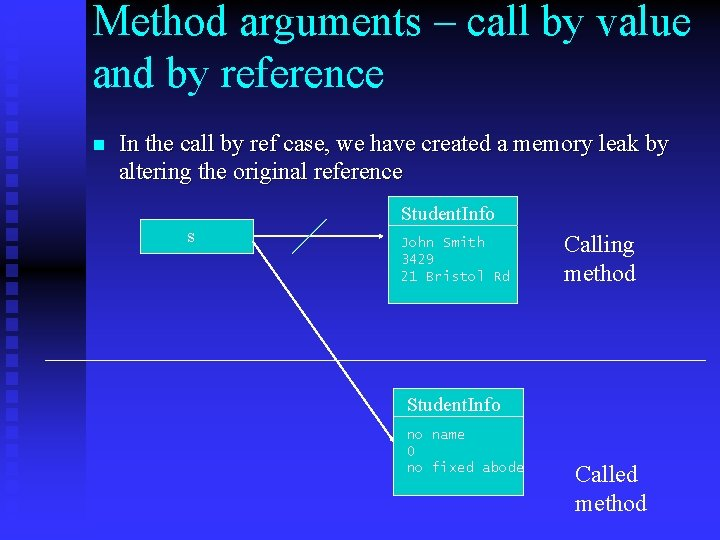Method arguments – call by value and by reference n In the call by ref case, we have created a memory leak by altering the original reference Student. Info s John Smith 3429 21 Bristol Rd Calling method Student. Info no name 0 no fixed abode Called method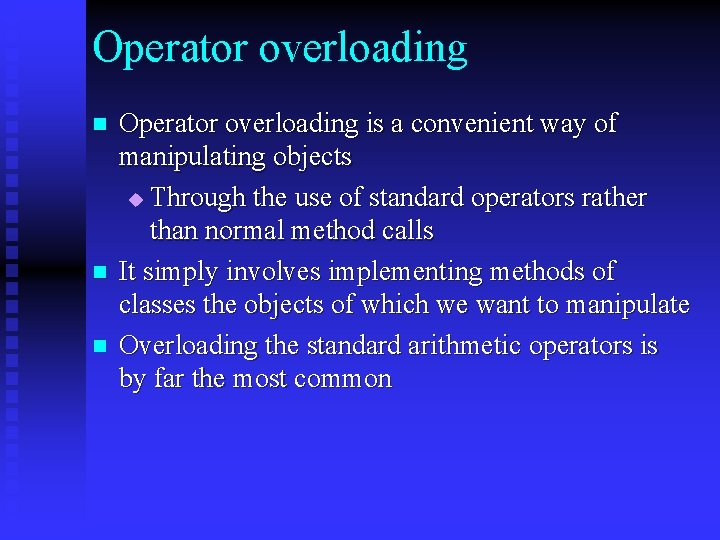Operator overloading n n n Operator overloading is a convenient way of manipulating objects u Through the use of standard operators rather than normal method calls It simply involves implementing methods of classes the objects of which we want to manipulate Overloading the standard arithmetic operators is by far the most common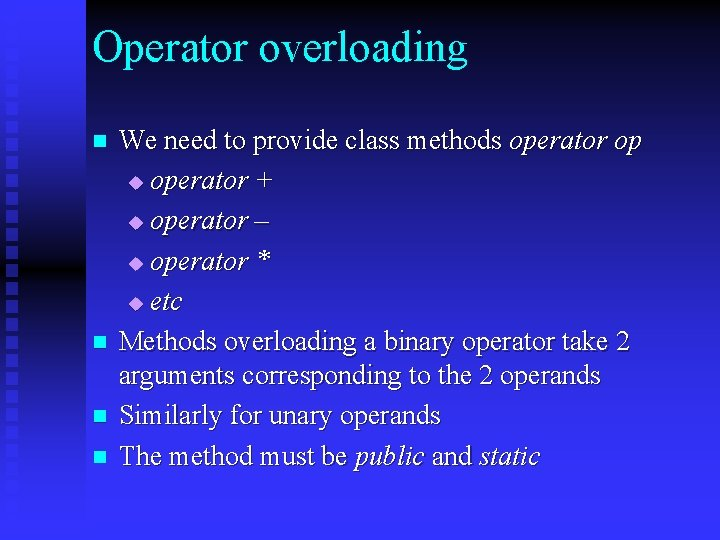Operator overloading n n We need to provide class methods operator op u operator + u operator – u operator * u etc Methods overloading a binary operator take 2 arguments corresponding to the 2 operands Similarly for unary operands The method must be public and static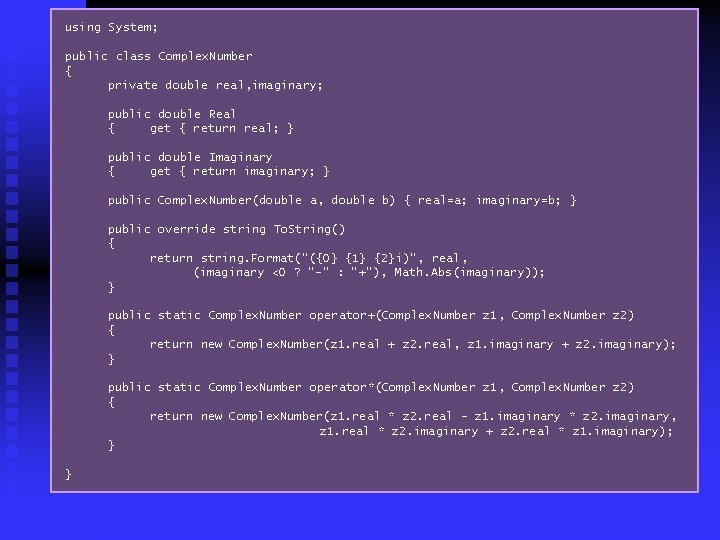using System; public class Complex. Number { private double real, imaginary; public double Real { get { return real; } public double Imaginary { get { return imaginary; } public Complex. Number(double a, double b) { real=a; imaginary=b; } public override string To. String() { return string. Format("({0} {1} {2}i)", real, (imaginary <0 ? "-" : "+"), Math. Abs(imaginary)); } public static Complex. Number operator+(Complex. Number z 1, Complex. Number z 2) { return new Complex. Number(z 1. real + z 2. real, z 1. imaginary + z 2. imaginary); } public static Complex. Number operator*(Complex. Number z 1, Complex. Number z 2) { return new Complex. Number(z 1. real * z 2. real - z 1. imaginary * z 2. imaginary, z 1. real * z 2. imaginary + z 2. real * z 1. imaginary); } }Operator overloading using System; public class Complex. Number. Test { static void Main(string[] args) { Complex. Number z 1, z 2; z 1 = new Complex. Number(1. 0, 2. 0); z 2 = new Complex. Number(3. 0, 5. 0); Complex. Number z 3 = z 1 + z 2; Complex. Number z 4 = z 1 * z 2; Console. Write. Line("{0} + {1}= {2}", z 1, z 2, z 3); Console. Write. Line("{0} * {1}= {2}", z 1, z 2, z 4); } }